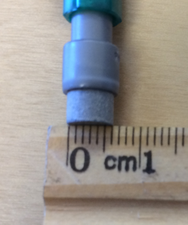# Unit Conversions

## Objective

Express decimal measurements in terms of a smaller unit.

## Common Core Standards

### Core Standards

?

• 4.MD.A.1 — Know relative sizes of measurement units within one system of units including km, m, cm; kg, g; lb, oz.; l, ml; hr, min, sec. Within a single system of measurement, express measurements in a larger unit in terms of a smaller unit. Record measurement equivalents in a two column table. For example, know that 1 ft is 12 times as long as 1 in. Express the length of a 4 ft snake as 48 in. Generate a conversion table for feet and inches listing the number pairs (1, 12), (2, 24), (3, 36), …

• 4.MD.A.2 — Use the four operations to solve word problems involving distances, intervals of time, liquid volumes, masses of objects, and money, including problems involving simple fractions or decimals, and problems that require expressing measurements given in a larger unit in terms of a smaller unit. Represent measurement quantities using diagrams such as number line diagrams that feature a measurement scale.

?

• 3.MD.A.1

• 3.MD.A.2

• 4.NF.C.5

• 4.NF.C.6

## Criteria for Success

?

1. Understand that 0.1 centimeter is equivalent to a millimeter.
2. Understand that 0.01 meter is equivalent to a centimeter.
3. Convert metric measurements from a larger unit written as a decimal to a smaller unit.

## Tips for Teachers

?

### Remote Learning Guidance

If you need to adapt or shorten this lesson for remote learning, we suggest prioritizing Anchor Task 2 (benefits from worked example). Find more guidance on adapting our math curriculum for remote learning here.

#### Fishtank Plus

Subscribe to Fishtank Plus to unlock access to additional resources for this lesson, including:

• Problem Set
• Student Handout Editor
• Google Classrom Integration
• Vocabulary Package

## Anchor Tasks

?

### Problem 1

Ms. Cole measures the width of her pencil eraser. She says it is 0.6 cm wide. Mr. Duffy says it is 6 mm wide. Who is correct? Explain your answer.#### Guiding Questions

Create a free account or sign in to access the Guiding Questions for this Anchor Problem.

### Problem 2

Solve. Show or explain your work.

a. 0.01 m = ________ cm

b. 0.06 m = ________ mm

c. 0.3 L = ________ mL

d. 0.83 kg = ________ g

e. 10.42 km = ________ m

#### Guiding Questions

Create a free account or sign in to access the Guiding Questions for this Anchor Problem.

## Problem Set & Homework

• Problem Set Answer Key
• Homework Answer Key

### Discussion of Problem Set

• How could your answer to #2 help you solve #4(a)?
• How could your answer to #3 help you solve #4(d)?
• How can knowing that 10 cm is equal to 100 mm help you check to make sure your answer to #4(c) is reasonable?
• Which cereal should Mr. Silver buy in #5? Why?
• How can knowing that 1 m is equal to 1,000 mm help you check to make sure your answer to #6(a) is reasonable?
• What is the combined length of Ms. Ruggiero’s ticker tape? Is it enough to use for the name tags? Why or why not?
• When have you heard someone talk about a decimal unit before?

## Target Task

?

### Problem 1

Show or explain how you know that 3.5 centimeters = 35 millimeters.

Create a free account or sign in to view Mastery Response

### Problem 2

Solve.

a.   4.8 meters = __________ millimeters

b.   5.04 L = __________ mL

c.   0.4 kg = __________ g

d.   10.67 km = __________ m

#### Mastery Response

Create a free account or sign in to view Mastery Response

## Additional Practice#### Unit Practice

With Fishtank Plus you can access our Daily Word Problem Practice and our content-aligned Fluency Activities created to help students strengthen their application and fluency skills.

View Preview